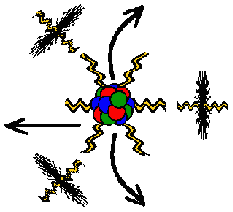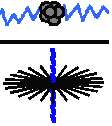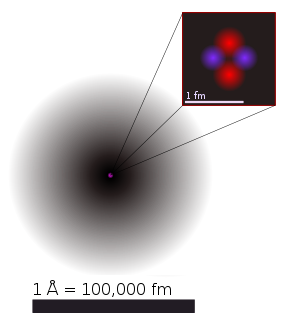Properties of Energy & Matter by Cranwell
 Nothing is Solid. Space is not Empty. Everything is Connected.

 Font Size A A A

 by James Clifford Cranwell 2/01/98 Part V ... Relativity

If there were only two objects in the universe (hypothetical), an observer and the Earth, both completely weightless / massless. The observer would be tricked into thinking he / she has weight because of the gravitational tug and mass because of the large concentration of gravitational tug. In their reality there are only forces. The Mass of the actual Flux particle is negligible... it is substantial only when it has a vibration or a gravitational pull on it. Therefore Mass is only a vibration or a pull, it isn't something we can actually take to the beach. Proof...

Note: some of these examples are intended only to show a direct correlation or relationship between everything ...inclusiveE = mc2 (Energy equals Mass times the speed of light squared) and...
E = hf (Energy equals frequency, h is constant).
Therefore, we can replace E in the first equation with hf and get the result hf = mc 2
When Mass is released as Energy it leaves the nucleus as Electrons traveling through free space at the speed of light, don't forget, the Electron is two dimensions. So, Energy equals Mass traveling at the speed (or length) of light in two dimensions or E = mc2
This can be thought of as the balled up strings of protons and neutrons (the mass) contained in the nucleus suddenly expanding, actually creating more of (what we call) space and vibrating away from center as 2-D electrons

A quick textbook type dimensional analysis gives even further insight...
The E or energy in this case is measured in joules with dimensions of [M][L2] / [T2]
That's mass times length squared divided by time squared
The m part of the mc2 is mass with dimensions of [M]
So far we have the energy part [M][L2] / [T2] = and the mass part [M]
To make the right side equal the left we have to multiply the mass by [L2] / [T2]
that's length2 divided by time2 or meters2/seconds2
Speed of light "c" is measured in meters per seconds [L] / [T]
Squaring this we arrive at the needed [L2] / [T2]
Is there anything that has the dimensional form [L2] / [T2] already?
What did the gravitational constant look like?.......m3 kg-1 s-2 = [L3] / [M][T2] units

In an atom, most of the Mass is contained in thenucleus. However, an Electron does have mass and the Proton / Electron mass ratio is 1836.1527556561 , As a result of this division we have canceled the strong force of light / gravity on four radii of the Electron-Proton combination in this example and we are left with 18 inanimate or inert radii of the Electron shell and the 18 curled radii of the Proton. Now we divide 1836.1527556561 by 10 square root26 (the exact value without the cancelled speed of light and gravity portion... negative one times speed of light, these were cancelled in the initial division) then divide by 36 (the number of curled radii and inert radii) and get the difference 1.0002755054517 ...This means everyone's measurements are correct to within two / ten thousandths and the ratio difference between 36 times 10 square root 26 and 1836.1527556561 is almost unity.

E = mc2 :
Energy equals Mass, speed of light squared is constant. As a result of the fusion reaction in the Sun, a tremendous amount of mass is converted to energy. Therefore, space is being filled with mass that has been converted or changed into energy. Since mass can be called particles and energy is the same as mass only in a different shape or state, we can say that space is filled with energy or particles, it isn't a void.
E = hf :
Energy equals frequency, h is constant. If we consider any point in space, we realize it has frequency or energy traversing it from every direction, this same process is happening at every point in space. Therefore every point in space contains energy or mass. Therefore if the Earth can be considered to have mass, space has an actual mass and cannot be a void.
E = hf = E = mc2 :
hf and mc2 are both equal to E (energy),
this means hf and mc2 are equal to each other ... hf = mc2.
h (plank) and c2 (Speed of light2) are dropable (ignorable) constants so,
f = m (frequency equals mass, everything has a frequency!), therefore frequency must have conveyance or a particle involved in its travels or else there will be nothing to contain energy when it converts back into mass. It works almost the same as electricity in wire. Particles are released from their mass or rest states and fill space at the speed of the solar wind. It is only the vibration effect conveyed on the particles that travels at the speed of light.Exact proton to atomic width size change:

The width of an atom is 100,000 times larger than the nucleus.
That makes the volumetric (spherical) difference of helium one quadrillion... 1,000,000,000,000,000

That means one quadrillion nucleus sized spheres can fit inside an atom sized sphere.
So if a proton converts from balled up to atom size, that is the exact change in size of the field at the spot. The amount of disruption or change in field size (commandeering) is from nucleus size to atom size.
This is the equivalent of energy (vibrations into the field) released by the shape change.
It would be like having one particle then having one quadrillion particles.

The check... E = mc^2 ...mass times speed of light is the amount.
And since everything is strings, and there are 20 string per particle, and there were 4 protons / neutrons in the nucleus (of this model).
Here is the answer (in meters per second)...

speed of light squared / one quadrillion = 89.8755179

And there are 20 strings per particle...
89.8755179 / 20 = 4.49377589

And there where 4 particles (proton, neutron) in the nucleus...
4.49377589 / 4 = 1.12344397

Since the answer is almost exactly one... it means if you divided the speed of light (squared) up into one quadrillion proton sized particles with 20 strings each they would almost exactly fit inside an atom sized sphere.

You have to understand what I mean by commandeering to understand this.
p.s. if you take sphere packing into account the answer might be even closer to one.
You have to remember the 100,000 (thousand) sized difference (although very accurate) is only an estimate.

Mass doesn't actually convert into energy... it changes shape.

Mass (a proton) changes in shape and that shape change vibrates the field... that is energy. The proton was made of string and they are still there.
It is kind of like if you had a guitar string with tension on it and added a millimeter instantly to the length... it would make the string vibrate.
They call it potential energy.

Quantum Weirdness:
The speed of light is only an effect created in the particle field. There shouldn't be any problem traveling faster than it. Except... every type of propulsion now uses something related to the same electron field vibration speed, for instance, chemical changes, nuclear reactions or even some type of electrical/magnetic propulsion would still be using the same electron vibrational speed. How can you travel/go faster than something that you have to use to go?
If you could travel fast enough to break free from field interactions you may find the speed of light incredibly slow (although there will be no way to discern this). A possible problem... once you have broken free from field interactions and are out of the space time continuum there is an absolute lack of reference frame and you become neutrino like with a speed and existence completely irrelevant to anything known. It may be impossible to re-enter or slow back into this space time.

 Properties of Energy & Matter * part VI *

Home Page    cranwell@gootar.com     https://xulfrepus.neocities.org/